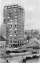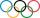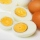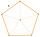# Fraction calculator

The calculator performs basic and advanced operations with fractions, expressions with fractions combined with integers, decimals, and mixed numbers. It also shows detailed step-by-step information about the fraction calculation procedure. Solve problems with two, three, or more fractions and numbers in one expression.

## Result:

### 62/3 - 43/4 = 23/12 = 1 11/12 ≅ 1.9166667

Spelled result in words is twenty-three twelfths (or one and eleven twelfths).

### How do you solve fractions step by step?

1. Conversion a mixed number 6 2/3 to a improper fraction: 6 2/3 = 6 2/3 = 6 · 3 + 2/3 = 18 + 2/3 = 20/3

To find new numerator:
a) Multiply the whole number 6 by the denominator 3. Whole number 6 equally 6 * 3/3 = 18/3
b) Add the answer from previous step 18 to the numerator 2. New numerator is 18 + 2 = 20
c) Write a previous answer (new numerator 20) over the denominator 3.

Six and two thirds is twenty thirds
2. Conversion a mixed number 4 3/4 to a improper fraction: 4 3/4 = 4 3/4 = 4 · 4 + 3/4 = 16 + 3/4 = 19/4

To find new numerator:
a) Multiply the whole number 4 by the denominator 4. Whole number 4 equally 4 * 4/4 = 16/4
b) Add the answer from previous step 16 to the numerator 3. New numerator is 16 + 3 = 19
c) Write a previous answer (new numerator 19) over the denominator 4.

Four and three quarters is nineteen quarters
3. Subtract: 20/3 - 19/4 = 20 · 4/3 · 4 - 19 · 3/4 · 3 = 80/12 - 57/12 = 80 - 57/12 = 23/12
For adding, subtracting, and comparing fractions, it is suitable to adjust both fractions to a common (equal, identical) denominator. The common denominator you can calculate as the least common multiple of both denominators - LCM(3, 4) = 12. In practice, it is enough to find the common denominator (not necessarily the lowest) by multiplying the denominators: 3 × 4 = 12. In the next intermediate step, the fraction result cannot be further simplified by canceling.
In words - twenty thirds minus nineteen quarters = twenty-three twelfths.

#### Rules for expressions with fractions:

Fractions - use the slash “/” between the numerator and denominator, i.e., for five-hundredths, enter 5/100. If you are using mixed numbers, be sure to leave a single space between the whole and fraction part.
The slash separates the numerator (number above a fraction line) and denominator (number below).

Mixed numerals (mixed fractions or mixed numbers) write as non-zero integer separated by one space and fraction i.e., 1 2/3 (having the same sign). An example of a negative mixed fraction: -5 1/2.
Because slash is both signs for fraction line and division, we recommended use colon (:) as the operator of division fractions i.e., 1/2 : 3.

Decimals (decimal numbers) enter with a decimal point . and they are automatically converted to fractions - i.e. 1.45.

The colon : and slash / is the symbol of division. Can be used to divide mixed numbers 1 2/3 : 4 3/8 or can be used for write complex fractions i.e. 1/2 : 1/3.
An asterisk * or × is the symbol for multiplication.
Plus + is addition, minus sign - is subtraction and ()[] is mathematical parentheses.
The exponentiation/power symbol is ^ - for example: (7/8-4/5)^2 = (7/8-4/5)2

#### Examples:

subtracting fractions: 2/3 - 1/2
multiplying fractions: 7/8 * 3/9
dividing Fractions: 1/2 : 3/4
exponentiation of fraction: 3/5^3
fractional exponents: 16 ^ 1/2
adding fractions and mixed numbers: 8/5 + 6 2/7
dividing integer and fraction: 5 ÷ 1/2
complex fractions: 5/8 : 2 2/3
decimal to fraction: 0.625
Fraction to Decimal: 1/4
Fraction to Percent: 1/8 %
comparing fractions: 1/4 2/3
multiplying a fraction by a whole number: 6 * 3/4
square root of a fraction: sqrt(1/16)
reducing or simplifying the fraction (simplification) - dividing the numerator and denominator of a fraction by the same non-zero number - equivalent fraction: 4/22
expression with brackets: 1/3 * (1/2 - 3 3/8)
compound fraction: 3/4 of 5/7
fractions multiple: 2/3 of 3/5
divide to find the quotient: 3/5 ÷ 2/3

The calculator follows well-known rules for order of operations. The most common mnemonics for remembering this order of operations are:
PEMDAS - Parentheses, Exponents, Multiplication, Division, Addition, Subtraction.
BEDMAS - Brackets, Exponents, Division, Multiplication, Addition, Subtraction
BODMAS - Brackets, Of or Order, Division, Multiplication, Addition, Subtraction.
GEMDAS - Grouping Symbols - brackets (){}, Exponents, Multiplication, Division, Addition, Subtraction.
Be careful, always do multiplication and division before addition and subtraction. Some operators (+ and -) and (* and /) has the same priority and then must evaluate from left to right.

## Fractions in word problems:

• The recipeThe recipe they are following requires 7/8 cups of milk, Tom already put 3/8 cups of milk. How much milk should Lea add to follow the recipe?
• KingKing had four sons. First inherit 1/2, second 1/4, third 1/5 of property. What part of the property was left to the last of the brothers?
• MichaelMichael had a bar of chocolate. He ate 1/2 of it and gave away 1/3. What fraction had he left?
• Hotel 4A 360 room hotel has 1/3 of its rooms occupied at present . How many rooms are empty?Pupils doing research which a winter sport do their classmates most popular. They found that 2/5 of classmates would most like to play hockey, skate prefer 2/9 pupils, 3/10 students prefer skiing and 1/15 classmates don't like any winter sport. What propo
• Claire and bookAfter Claire has read the first 5/8 of the book, there are 120 pages left to read. What is the total number of pages of the book?
• ShirtsIn a classroom, 1/6 of the students are wearing blue shirts and 2/3 are wearing white shirts. There are 36 students in the class. How many students are wearing a shirt other than blue or white?
• 30 eggsThere are 30 eggs in a tray. If 1/2 of the tray used 1/5 of it cooked,1/3 kept the refrigerator, how many eggs were left?Sadie practiced her spelling words for 3/4 of an hour, and Max practiced his spelling words for 5/12 of an hour. In the simplest form, how much longer did Sadie practice than Max?Bitoo ate 3/5 part of an apple and the remaining part was eaten by his sister Reena. How much part of an apple did Renna eat? Who had the larger share? By how much?This is algebra. Let n represent an unknown number. 1. Eight more than the number n 2. Three times the number n 3. The product of the number n and eight 4. Three less than the number n 5. Three decreased by the number nOf the shapes,1/6 are triangles and 5/12 are pentagons. What fraction of the shapes are either triangles or pentagons?Ali bought 5/6 litre of milk. He drank 1/2 litre and his brother drank 1/6 litre. How much litre of milk left?